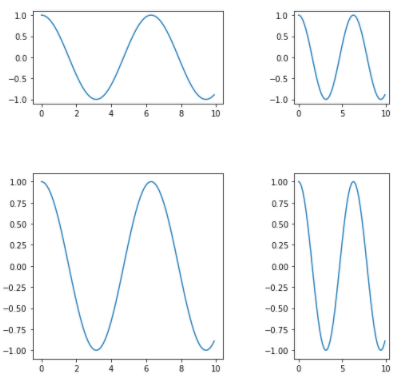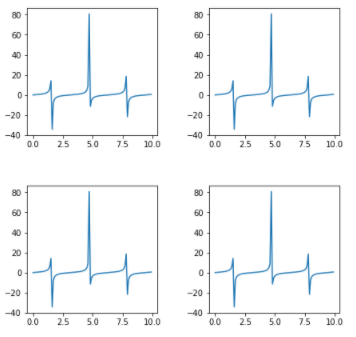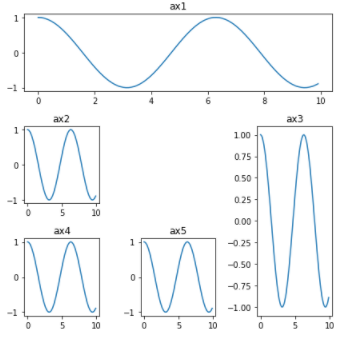# How to Create Different Subplot Sizes in Matplotlib?

In this article, we will learn different ways to create subplots of different sizes using Matplotlib. It provides 3 different methods using which we can create different subplots of different sizes.

Methods available to create subplot:

• Gridspec
• gridspec_kw
• subplot2grid

## Create Different Subplot Sizes in Matplotlib using Gridspec

The GridSpec from the gridspec module is used to adjust the geometry of the Subplot grid. We can use different parameters to adjust the shape, size, and number of columns and rows.

gridspec.GridSpec(ncols=2, nrows=2, width_ratios=[2, 1],wspace=0.5,hspace=0.5,height_ratios=[1,2])

## Python3

 `# importing required libraries` `import` `matplotlib.pyplot as plt` `from` `matplotlib ``import` `gridspec` `import` `numpy as np`   `# create a figure` `fig ``=` `plt.figure()`   `# to change size of subplot's` `# set height of each subplot as 8` `fig.set_figheight(``8``)`   `# set width of each subplot as 8` `fig.set_figwidth(``8``)`   `# create grid for different subplots` `spec ``=` `gridspec.GridSpec(ncols``=``2``, nrows``=``2``,` `                         ``width_ratios``=``[``2``, ``1``], wspace``=``0.5``,` `                         ``hspace``=``0.5``, height_ratios``=``[``1``, ``2``])`   `# initializing x,y axis value` `x ``=` `np.arange(``0``, ``10``, ``0.1``)` `y ``=` `np.cos(x)`   `# ax0 will take 0th position in ` `# geometry(Grid we created for subplots),` `# as we defined the position as "spec"` `ax0 ``=` `fig.add_subplot(spec[``0``])` `ax0.plot(x, y)`   `# ax1 will take 0th position in` `# geometry(Grid we created for subplots),` `# as we defined the position as "spec"` `ax1 ``=` `fig.add_subplot(spec[``1``])` `ax1.plot(x, y)`   `# ax2 will take 0th position in` `# geometry(Grid we created for subplots),` `# as we defined the position as "spec"` `ax2 ``=` `fig.add_subplot(spec[``2``])` `ax2.plot(x, y)`   `# ax3 will take 0th position in` `# geometry(Grid we created for subplots),` `# as we defined the position as "spec"` `ax3 ``=` `fig.add_subplot(spec[``3``])` `ax3.plot(x, y)`   `# display the plots` `plt.show()`

Output:## Create Different Subplot Sizes in Matplotlib gridspec_kw

It is a dictionary available inside “plt.subplots()” method in Matplotlib. By passing different parameters to the dictionary we can adjust the shape and size of each subplot.

plt.subplots(nrows=2, ncols=2,figsize=(7,7), gridspec_kw={‘width_ratios’: [3, 3], ‘height_ratios’: [3, 3], ‘wspace’ : 0.4, ‘hspace’ : 0.4})

Code :

## Python3

 `# importing required libraries` `import` `matplotlib.pyplot as plt` `import` `numpy as np`   `# setting different parameters to adjust each grid` `fig, ax ``=` `plt.subplots(nrows``=``2``, ncols``=``2``, figsize``=``(``7``, ``7``),` `                       ``gridspec_kw``=``{` `                           ``'width_ratios'``: [``3``, ``3``],` `                           ``'height_ratios'``: [``3``, ``3``],` `                       ``'wspace'``: ``0.4``,` `                       ``'hspace'``: ``0.4``})`     `# initializing x,y axis value` `x ``=` `np.arange(``0``, ``10``, ``0.1``)` `y ``=` `np.tan(x)`   `# ax will take 0th position in ` `# geometry(Grid we created for subplots)` `ax[``0``][``0``].plot(x, y)`   `# ax will take 0th position in` `# geometry(Grid we created for subplots)` `ax[``0``][``1``].plot(x, y)`   `# ax will take 0th position in` `# geometry(Grid we created for subplots)` `ax[``1``][``0``].plot(x, y)`   `# ax will take 0th position in` `# geometry(Grid we created for subplots)` `ax[``1``][``1``].plot(x, y)`   `plt.show()`

Output :## Create Different Subplot Sizes in Matplotlib subplot2grid

It provides more flexibility to create a grid at any location. We can extend the grid horizontally as well as vertically very easily. Here shape is denoting to no. of rows and columns and loc denotes the geometric location of the grid. Whereas, colspan = 3 in ax1, it means this subplot will cover 3 of all columns of that row. If we give irregular input then it will give an error so we have given the proper value to colspan and rowspan.

plt.subplot2grid(shape=(3, 3), loc=(0, 0), colspan=3)

## Python3

 `# importing required library` `import` `matplotlib.pyplot as plt` `import` `numpy as np`   `# creating grid for subplots` `fig ``=` `plt.figure()` `fig.set_figheight(``6``)` `fig.set_figwidth(``6``)`   `ax1 ``=` `plt.subplot2grid(shape``=``(``3``, ``3``), loc``=``(``0``, ``0``), colspan``=``3``)` `ax2 ``=` `plt.subplot2grid(shape``=``(``3``, ``3``), loc``=``(``1``, ``0``), colspan``=``1``)` `ax3 ``=` `plt.subplot2grid(shape``=``(``3``, ``3``), loc``=``(``1``, ``2``), rowspan``=``2``)` `ax4 ``=` `plt.subplot2grid((``3``, ``3``), (``2``, ``0``))` `ax5 ``=` `plt.subplot2grid((``3``, ``3``), (``2``, ``1``), colspan``=``1``)`     `# initializing x,y axis value` `x ``=` `np.arange(``0``, ``10``, ``0.1``)` `y ``=` `np.cos(x)`   `# plotting subplots` `ax1.plot(x, y)` `ax1.set_title(``'ax1'``)` `ax2.plot(x, y)` `ax2.set_title(``'ax2'``)` `ax3.plot(x, y)` `ax3.set_title(``'ax3'``)` `ax4.plot(x, y)` `ax4.set_title(``'ax4'``)` `ax5.plot(x, y)` `ax5.set_title(``'ax5'``)`   `# automatically adjust padding horizontally ` `# as well as vertically.` `plt.tight_layout()`   `# display plot` `plt.show()`

Output :Whether you're preparing for your first job interview or aiming to upskill in this ever-evolving tech landscape, GeeksforGeeks Courses are your key to success. We provide top-quality content at affordable prices, all geared towards accelerating your growth in a time-bound manner. Join the millions we've already empowered, and we're here to do the same for you. Don't miss out - check it out now!

Previous
Next